# Jean Léonard Marie Poiseuille facts for kids

Kids Encyclopedia Facts
Quick facts for kids
Jean Léonard Marie Poiseuille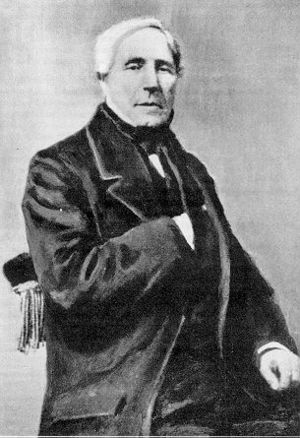Born 22 April 1797
Died 26 December 1869 (aged 72)
Nationality French
Alma mater École Polytechnique
Known for Poiseuille's law
Scientific career
Fields physicist and physiologist

Jean Léonard Marie Poiseuille ( 22 April 1797 – 26 December 1869) was a French physicist and physiologist.

Poiseuille was born in Paris, France, and he died there on 26 December 1869.

## Fluid flow

From 1815 to 1816 he studied at the École Polytechnique in Paris. He was trained in physics and mathematics. In 1828 he earned his D.Sc. degree with a dissertation entitled Recherches sur la force du coeur aortique. He was interested in the flow of human blood in narrow tubes.

In 1838 he experimentally derived, and in 1840 and 1846 formulated and published, Poiseuille's law (now commonly known as the Hagen–Poiseuille equation, crediting Gotthilf Hagen as well), which applies to laminar flow, that is, non-turbulent flow of liquids through pipes of uniform section, such as blood flow in capillaries and veins. His original formulation for water of 1846 little resembles the present-day formulation and is given as: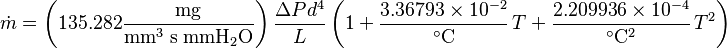$\dot{m} = \left(135.282 \mathrm{\frac{mg}{ mm^3\; s \; mm H_2O}} \right) \frac{\Delta P d^4}{L} \left(1+\frac{3.36793\times10^{-2}}{^\circ \mathrm{C}}\,T +\frac{2.209936\times10^{-4}}{^\circ \mathrm{C}^2}\,T^2\right)$

However it can be transformed in a more amenable form. Rewritting in more modern fashion using SI units gives: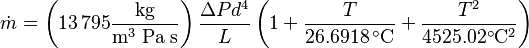$\dot{m} = \left(13\,795 \mathrm{\frac{kg}{ m^3\; Pa \; s}} \right) \frac{\Delta P d^4}{L} \left(1+\frac{T}{26.6918\, ^\circ \mathrm{C}} +\frac{T^2}{4525.02^\circ \mathrm{C}^2}\right)$

Using the density of water as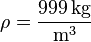$\mathrm{\rho=\frac{999\,kg}{m^3}}$ and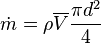$\dot{m}= \rho\overline{V}\frac{\pi d^2}{4}$ and then solving for the pressure difference results in: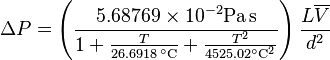$\Delta P =\left(\frac{5.68769 \times10^{-2} \mathrm{Pa\,s}} {1+\frac{T}{26.6918\, ^\circ \mathrm{C}} +\frac{T^2}{4525.02^\circ \mathrm{C}^2}}\right) \frac{L\overline{V}}{d^2}$

The term in parentheses, the constant and the temperature correction, are a function of viscosity. Finally using the viscosity of water at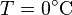$T= 0 ^\circ \mathrm{C}$,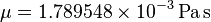$\mu = 1.789548 \times10^{-3}\,\mathrm{Pa\,s}$, allows for viscosity of different fluids to be taken into account resulting in: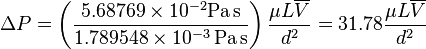$\Delta P = \left(\frac{5.68769 \times10^{-2} \mathrm{Pa\,s}}{1.789548 \times10^{-3}\,\mathrm{Pa\,s}}\right) \frac{\mu L\overline{V}}{d^2} = 31.78 \frac{\mu L \overline{V}}{d^2}$

The equation in standard fluid dynamics notation is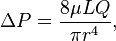$\Delta P = \frac{8 \mu L Q}{\pi r^4},$

or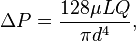$\Delta P = \frac{128 \mu L Q}{\pi d^4},$

or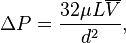$\Delta P = \frac{32 \mu L \overline{V}}{d^2},$

where: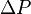$\Delta P$ is the pressure loss,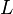$L$ is the length of pipe,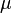$\mu$ is the dynamic viscosity,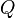$Q$ is the volumetric flow rate,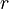$r$ is the radius,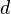$d$ is the diameter,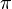$\pi$ is the mathematical constant π,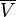$\overline{V}$ is the average velocity.

The poise, the unit of viscosity in the CGS system, was named after him. Attempts to introduce "poiseuille" as the name of the SI unit Pa·s had little success.Jean Léonard Marie Poiseuille Facts for Kids. Kiddle Encyclopedia.